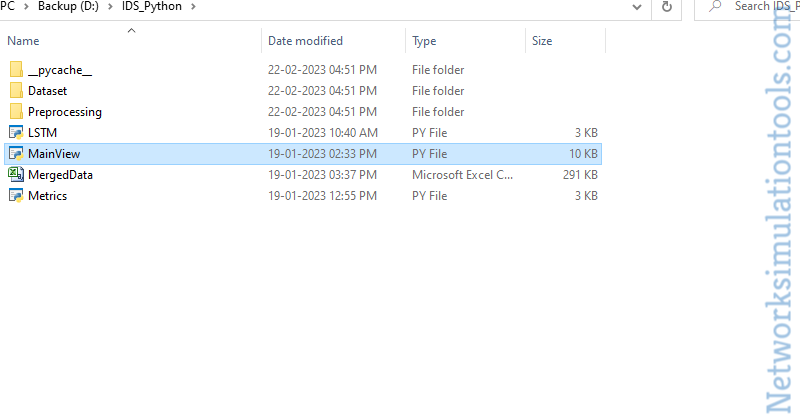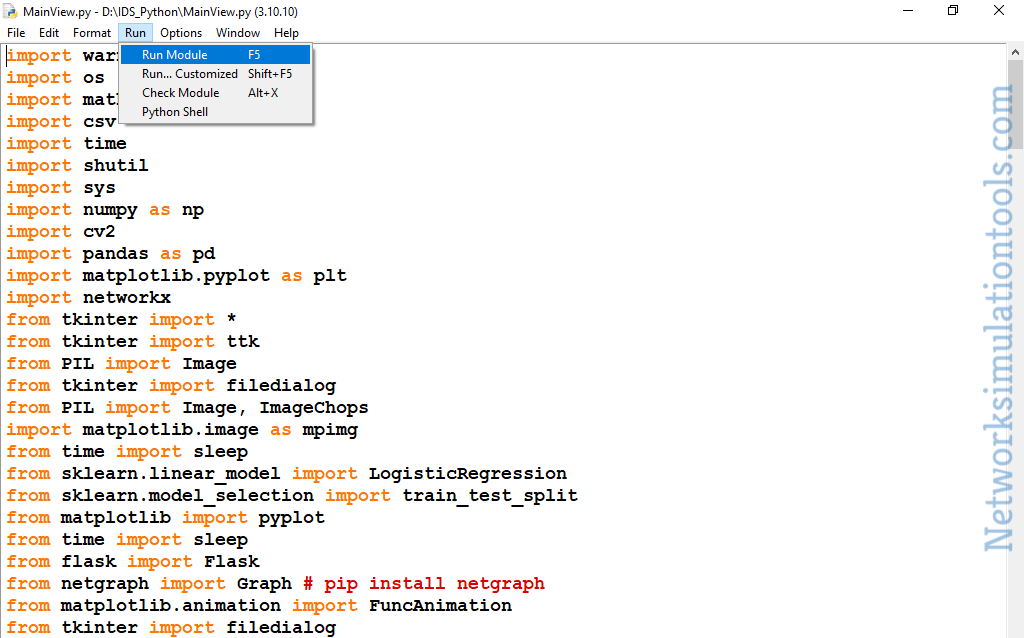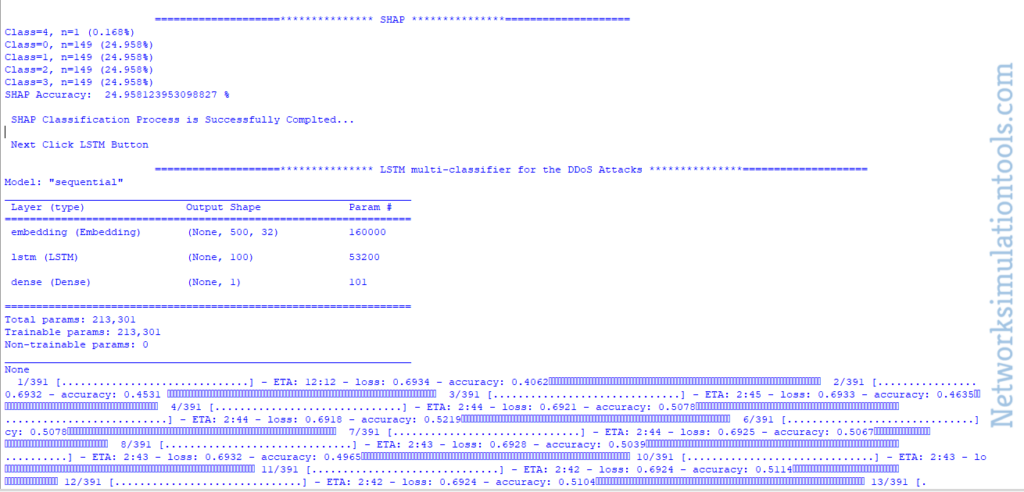# How to Implement the Intrusion Detection System in Python

How to Implement the Intrusion Detection System in Python

To answer this question “how to implement the intrusion detection system in python”, our technical professionals have described this article. So, make use of it to grab the answers.

Create Python File for IDS

As the first process, we have to create the python main file to perform the intrusion detection system process for that the main file has to be created along with the file extension .py as shown in the following image.Python Code for Intrusion Detection System

Additionally, we have highlighted the python based sample code for the process such as classification and detection of intrusions.

``` def Multiclass_Classification_Shap(): time.sleep(3) print ("\n\t\t\t====================*************** SHAP ***************====================") from pandas import read_csv from collections import Counter from matplotlib import pyplot from sklearn.preprocessing import LabelEncoder # define the dataset location url = 'MergedData.csv' acc = 65; # load the csv file as a data frame df = read_csv(url, header=None) data = df.values # split into input and output elements X, y = data[:, :-1], data[:, -1] # label encode the target variable y = LabelEncoder().fit_transform(y) # summarize distribution counter = Counter(y) for k,v in counter.items(): per = v / len(y) * 100 print('Class=%d, n=%d (%.3f%%)' % (k, v, per)) # plot the distribution pyplot.bar(counter.keys(), counter.values()) pyplot.show() print("SHAP Accuracy: ",str(per),"%") print ("\n SHAP Classification Process is Successfully Completed...") print ("\n Next Click LSTM Button") def lstm(): time.sleep(3) print ("\n\t\t\t====================*************** LSTM multi-classifier for the DDoS Attacks ***************====================") import tensorflow as tf from tensorflow.keras.datasets import imdb from tensorflow.keras.models import Sequential from tensorflow.keras.layers import Dense from tensorflow.keras.layers import LSTM from tensorflow.keras.layers import Embedding from tensorflow.keras.preprocessing import sequence # fix random seed for reproducibility tf.random.set_seed(7) # load the dataset but only keep the top n words, zero the rest top_words = 5000 (X_train, y_train), (X_test, y_test) = imdb.load_data(num_words=top_words) # truncate and pad input sequences max_review_length = 500 X_train = sequence.pad_sequences(X_train, maxlen=max_review_length) X_test = sequence.pad_sequences(X_test, maxlen=max_review_length) # create the model embedding_vecor_length = 32 model = Sequential() model.add(Embedding(top_words, embedding_vecor_length, input_length=max_review_length)) model.add(LSTM(100)) model.add(Dense(1, activation='sigmoid')) model.compile(loss='binary_crossentropy', optimizer='adam', metrics=['accuracy']) print(model.summary()) model.fit(X_train, y_train, epochs=1, batch_size=64) # Final evaluation of the model scores = model.evaluate(X_test, y_test, verbose=0) print("Accuracy: %.2f%%" % (scores*100)) print ("\n LSTM is Successfully Completed...") print ("\n Next Click PERFORMANCE METRICS Button") ```

IDS Implementation Using Python

We have to go to the menu and click the run module option to implement intrusion detection system using python.As an additional note, we have highlighted the results that are executed over the intrusion detection using python.If you guys have any issues while implementing the intrusion detection system in python, without any hesitation you guys can contact us.

Technology Ph.D MS M.Tech
NS2 75 117 95
NS3 98 119 206
OMNET++ 103 95 87
OPNET 36 64 89
QULANET 30 76 60
MININET 71 62 74
MATLAB 96 185 180
LTESIM 38 32 16
COOJA SIMULATOR 35 67 28
CONTIKI OS 42 36 29
GNS3 35 89 14
NETSIM 35 11 21
EVE-NG 4 8 9
TRANS 9 5 4
PEERSIM 8 8 12
GLOMOSIM 6 10 6
RTOOL 13 15 8
VNX and VNUML 8 7 8
WISTAR 9 9 8
CNET 6 8 4
ESCAPE 8 7 9
NETMIRAGE 7 11 7
BOSON NETSIM 6 8 9
VIRL 9 9 8
CISCO PACKET TRACER 7 7 10
SWAN 9 19 5
JAVASIM 40 68 69
SSFNET 7 9 8
TOSSIM 5 7 4
PSIM 7 8 6
PETRI NET 4 6 4
ONESIM 5 10 5
OPTISYSTEM 32 64 24
DIVERT 4 9 8
TINY OS 19 27 17
TRANS 7 8 6
OPENPANA 8 9 9
SECURE CRT 7 8 7
EXTENDSIM 6 7 5
CONSELF 7 19 6
ARENA 5 12 9
VENSIM 8 10 7
MARIONNET 5 7 9
NETKIT 6 8 7
GEOIP 9 17 8
REAL 7 5 5
NEST 5 10 9
PTOLEMY 7 8 4